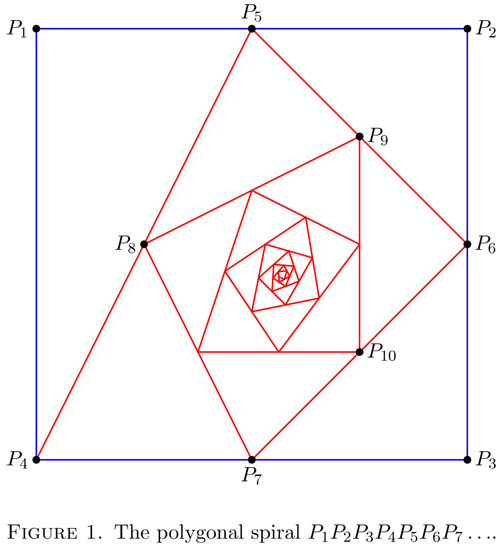# Problem of the Week #78 - September 23rd, 2013

Status
Not open for further replies.

#### Chris L T521

##### Well-known member
Staff member
Thanks again to those who participated in last week's POTW! Here's this week's problem!

-----

Problem: Starting with the vertices $P_1(0,1)$, $P_2(1,1)$, $P_3(1,0)$, $P_4(0,0)$ on a square, we construct further points as shown in the figure below: $P_5$ is the midpoint of $P_1P_2$, $P_6$ is the midpoint of $P_2P_3$, $P_7$ is the midpoint of $P_3P_4$, and so on. The polygonal spiral path $P_1P_2P_3P_4P_5P_6P_7\ldots$ approaches a point $P$ inside the square.a) If the coordinates of $P_n$ are $(x_n,y_n)$, show that $\frac{1}{2}x_n + x_{n+1} + x_{n+2} + x_{n+3} = 2$ and find a similar equation for the $y$-coordinates.

b) Find the coordinates of $P$.

-----

#### Chris L T521

##### Well-known member
Staff member
This week's problem was correctly answered by MarkFL. You can find his answer below.
Let's generalize a little to use a rectangle of base $b$ and height $h$ instead of a unit square, hence:

$$\displaystyle P_1=(0,h),\,P_2=(b,h),\,P_3=(b,0),\,P_4=(0,0)$$

a) We see that both $x_n$ and $y_n$ may be found using the recursive algorithm:

$$\displaystyle u_{n+4}\equiv\frac{u_{n}+u_{n+1}}{2}$$

This follows from the mid-point formula. Now, for such an algorithm, consider the following sum:

$$\displaystyle u_{n+4}+2u_{n+5}+2u_{n+6}+2_{n+7}=k$$

Using the algorithm, we have the following:

$$\displaystyle u_{n+4}+u_{n+1}+u_{n+2}+u_{n+2}+u_{n+3}+u_{n+3}+u_{n+4}=k$$

Collecting like terms, we find:

$$\displaystyle u_{n+1}+2u_{n+2}+2u_{n+3}+2u_{n+4}=k$$

From this, we may therefore conclude that for any $n$, we have:

$$\displaystyle u_{n}+2u_{n+1}+2u_{n+2}+2u_{n+3}=k$$

Where the parameter $k$ may be determined from the first 4 values of the sequence.

For the sequence $x_{n}$, we find:

$$\displaystyle x_{1}+2x_{2}+2x_{3}+2x_{4}=4b\implies \frac{1}{2}x_{n}+x_{n+1}+x_{n+2}+x_{n+3}=2b$$

For the sequence $y_n$, we find:

$$\displaystyle y_{1}+2y_{2}+2y_{3}+2y_{4}=3h\implies \frac{1}{2}y_{n}+y_{n+1}+y_{n+2}+y_{n+3}=\frac{3h}{2}$$

Using the data given for this problem:

$$\displaystyle b=h=1$$

we have:

$$\displaystyle \frac{1}{2}x_{n}+x_{n+1}+x_{n+2}+x_{n+3}=2$$

$$\displaystyle \frac{1}{2}y_{n}+y_{n+1}+y_{n+2}+y_{n+3}=\frac{3}{2}$$

b) Since the coordinates are converging to one value, namely $P(x,y)$, we may write:

$$\displaystyle x+2x+2x+2x=7x=4b\implies x=\frac{4b}{7}$$

$$\displaystyle y+2y+2y+2y=7y=3h\implies y=\frac{3h}{7}$$

Hence:

$$\displaystyle P(x,y)=\left(\frac{4b}{7},\frac{3h}{7} \right)$$

Using the data given for this problem:

$$\displaystyle b=h=1$$

we have:

$$\displaystyle P(x,y)=\left(\frac{4}{7},\frac{3}{7} \right)$$

edit by MarkFL (with permission given by Chris L T521):

The question about convergence of the sequence was brought up by one of our members. You can find the proof of convergence below:

Consider the linear homogenous recurrence obtained from the mid-point formula:

$$\displaystyle u_{n+4}=\frac{u_{n}+u_{n+1}}{2}$$

We may write this as:

$$\displaystyle 2u_{n+4}=u_{n+1}+u_{n}$$

From which, we obtain the characteristic polynomial:

$$\displaystyle 2r^4-r-1=(r-1)\left(2r^3+2r^2+2r+1 \right)=0$$

The characteristic roots, the irrational of which I will approximate, are given by:

$$\displaystyle r\approx1,\,-0.647798871261042,\, -0.176100564369479\pm0.860716618623568i$$

Hence, the closed form can be approximated with:

$$\displaystyle u_{n}\approx k_1+k_2(-0.647798871261042)^n+k_3(0.878546815113479)^n \sin\left(n\theta+k_4 \right)$$

We see then that we must have:

$$\displaystyle \lim_{n\to\infty}u_{n}=k_1$$

That is, the sequence $u_{n}$ must converge to some fixed value.

Status
Not open for further replies.